## What is the radius? The radius is the distance from the centre of a circle to the outer edge of a circle.

This is shown in the diagram below: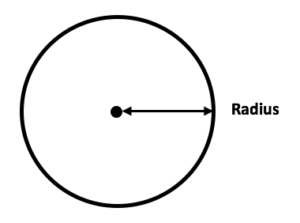Knowing the radius of a circle means you can also work out the diameter, as the diameter is the distance right across the centre of a circle. The diameter is always twice the length of the radius.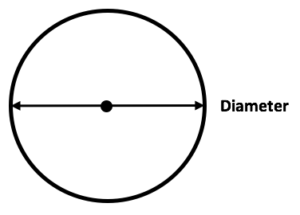After learning about 2D and 3D shapes in Years 1 and 2, children will be introduced to the different parts of a circle in Year 6.

Teachers will ask children to measure the radius and diameter of different circles and may also give them string so that they can measure the circumference of the circle.

## What questions will children be given involving the radius?

Once they have learnt that the diameter of a circle is twice the radius, children might be introduced to the formula ‘C= 2 x π x r’, which will help them calculate the circumference of a circle more accurately. Here is an example of how to use the formula in a question: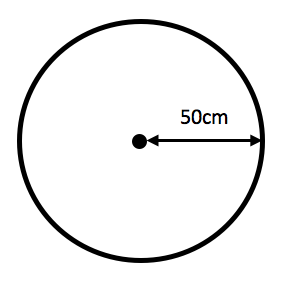Question: Calculate the circumference of this circle, where π = 3.14.

We are told that the radius of the circle is 50cm, so we can now substitute this into the formula C  =  2 x π x r:

2 x 3.14 x 50 = 314 cm

Circumference = 314 cm

If the diagram only gave the diameter of the circle, then children could either half the diameter to find the radius, or use the formula C  =  π x d.

Teachers may also give children the formula for working out the area of a circle:  π x r ². This can be used like so: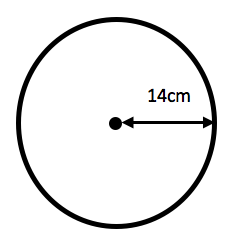Question: Calculate the area of this circle, where π = 3.14.

We are told that the radius of the circle is 14cm, so we can now substitute this into the formula A  =  π x r ²:

3.14 x 14 x 14 = 615.44 cm²

Area = 615.44 cm² (units of area are always written as squares, such as cm² and m²).

## How to help children with the radius?

It is important that children spend enough time learning the definitions of different parts of a circle, so that they do not get them confused when doing practice questions. Having access to a labelled diagram of a circle can help children build up confidence when they are first learning the definitions.

Sometimes children will be given practice questions which are scenario based and that do not have diagrams drawn. For these questions, it is helpful to encourage children to draw a diagram out themselves, so that they can visualise what the question is expecting them to do.

For example:

Rebecca has a circular pond in her back garden. The distance from the middle of the pond to the edge is 145 cm. What is the circumference of the pond?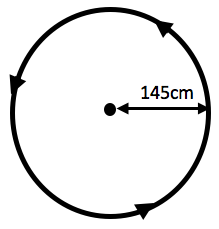Answer:

First, draw out a circle for the pond and label it with the measurements given.

Then to work out circumference use the formula 2 x π x r (where π = 3.14):

2 x 3.14 x 145 = 910.6 cm

The circumference of the pond is 910.6 cm

## How does Learning Street help children with the radius?

Our specialised maths courses will introduce the radius to the child at the same time as they learn about it at school in order to aid their learning. They will be taught about it and then have questions and exercises to practice on in order that they can do it in exams.

Tests could include SATs, competitive 11 Plus tests or selective Independent school exams.

## Our Courses

Click through to review the courses we have available

##### Get Started

Learning Street structured courses make home study easier and more successful. There's no need to wonder which books to buy or what to do next. Everything is planned for you.

• Proven to deliver excellent results
• Used by families, private tutors and schools
• Fully planned and structured